Next: Shell knot Up: Mathematical description of a Previous: Mathematical description of a   Contents

#### Beam knot

The expansion of a single beam node leads to a planar set of nodes. Therefore, the stretch of a knot based on this expansion is reduced to the stretch along the two principal directions in that plane. The stretch in the direction of the beam axis is not relevant. Let us assume that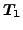is a unit vector tangent to the local beam axis and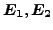are two unit vectors in the expansion plane such that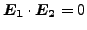and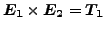. Then, the stretch in the plane can be characterized by vectors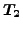and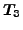along its principal directions: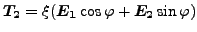(148)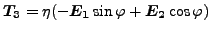(149)

leading to three stretch degrees of freedom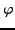,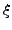and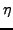.is the anglemakes with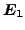,is the stretch alongandis the stretch along. The right stretch tensor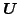can now be written as: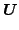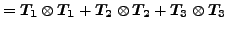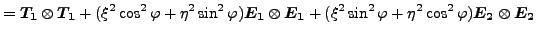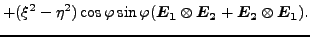(150)

The rotation vector reads in component notation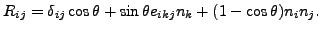(151)

Here,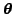is a vector along the rotation axis satisfying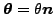,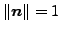. Assuming that at some point in the calculation the knot is characterized by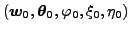, a change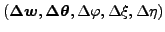leads to (cf. Equation (146)):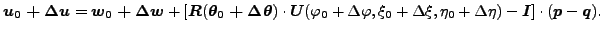(152)

Taylor expansion of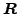: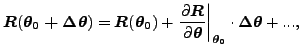(153)

and similar forand keeping linear terms only leads to the following equation: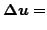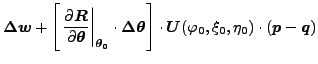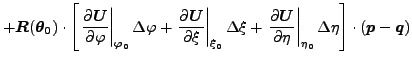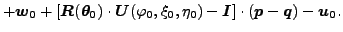(154)

The latter equation is a inhomogeneous linear equation linking the change in displacements of an arbitrary node belonging to a knot to the change in the knot parameters (translation, rotation and stretch). This equation is taken into account at the construction phase of the governing equations. In that way the expanded degrees of freedom, being dependent, never show up in the equations to solve.Next: Shell knot Up: Mathematical description of a Previous: Mathematical description of a   Contents
guido dhondt 2018-12-15